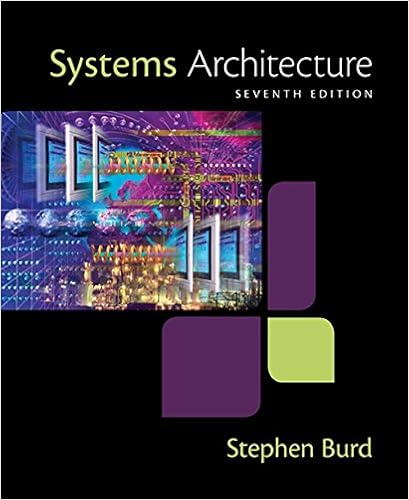# Deal five blackjackcard s each to the player and the

• Homework Help
• 47
• 97% (172) 167 out of 172 people found this document helpful

This preview shows page 43 - 46 out of 47 pages.

##### We have textbook solutions for you!
The document you are viewing contains questions related to this textbook.The document you are viewing contains questions related to this textbook.
Chapter 13 / Exercise 18
Systems Architecture
BurdExpert Verified
total value without exceeding 21. Deal five BlackjackCards each to the player and the computer. Make sure that each BlackjackCardis unique. For example, a deck cannot contain more than one Queen of Spades.Determine the winner as follows:If the player’s first two, first three, first four, or all five cards have a total value of exactly 21, the player wins, even if the computer also achieves a total of 21.If the player’s first two cards do not total exactly 21, sum as many as needed to achieve the highest possible total that does not exceed 21. For example, suppose the player’s five cards are valued as follows: 10, 4, 5, 9, 2. In that case, the player’s total for the first three cards is 19; counting any more cards would cause the total to exceed 21.After you have determined the player’s total, sum the computer’s cards in sequence. For example, suppose the computer’s cards are 10, 10, 5, 6, 7. The first two cards total 20; you would not use the third card because it would cause the total to exceed 21.The winner has the highest total among the cards used. For example, if the player’s total using the first three cards is 19 and the computer’s total using the
##### We have textbook solutions for you!
The document you are viewing contains questions related to this textbook.The document you are viewing contains questions related to this textbook.
Chapter 13 / Exercise 18
Systems Architecture
BurdExpert Verified
Programming Logic and Design, 8eSolutions 11-44first two cards is 20, the computer wins.Display a message that indicates whether the game ended in a tie, the computer won, or the player won.
Programming Logic and Design, 8eSolutions 11-45// check valuesx = 0playerSum = 0playerDone = 0playerWin = 0while x < NUM_OF_CARDS AND playerDone = 0playerSum = playerCards[x] + playerSumif playerSum = WINNING_NUMBER thenplayerWin = 1playerDone = 1elseif playerSum > WINNING_NUMBER thenplayerSum = playerSum – playerCards[x]playerDone = 1endifendifx = x + 1endwhile// check valuesx = 0computerSum = 0computerDone = 0computerWin = 0while x < NUM_OF_CARDS AND computerDone = 0 AND playerWin <> 1computerSum = computerCards[x] + computerSumif computerSum = WINNING_NUMBER thencomputerWin = 1computerDone = 1else
•••